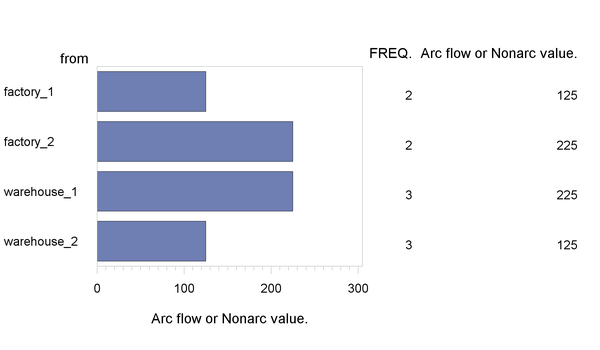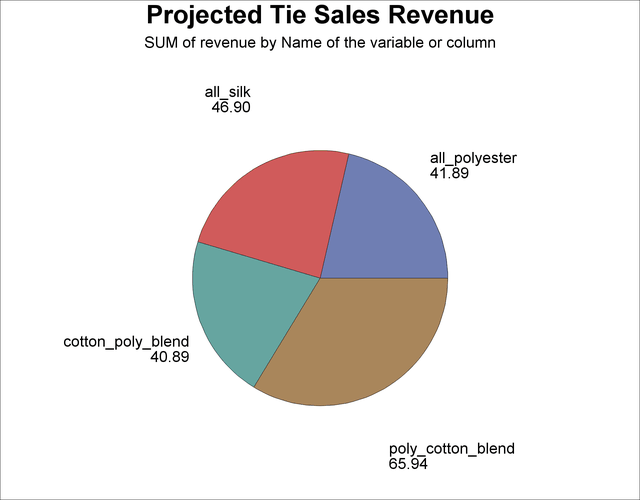### Other Reporting Procedures

The GCHART procedure can be a useful tool for displaying the solution to mathematical programming models. The `con_solv` data set that contains the solution to the balanced transshipment problem can be effectively displayed using PROC GCHART. In Figure 3.10, the amount that is shipped from each factory and warehouse can be seen by submitting the following SAS statements:

```title;
proc gchart data=con_sav;
hbar from / sumvar=_flow_;
run;
```

Figure 3.10: Tie Problem: ThroughputsThe horizontal bar chart is just one way of displaying the solution to a mathematical program. The solution to the tie product mix problem that was solved using PROC LP can also be illustrated using PROC GCHART.

```proc gchart data=product;
pie _var_ / sumvar=revenue;
title h=2.5 'Projected Tie Sales Revenue';
run;
```

The pie chart in Figure 3.11 shows the relative contribution of each product to total revenues.

Figure 3.11: Tie Problem: Projected Tie Sales RevenueThe TABULATE procedure can also help automate solution reporting. Several examples in Chapter 5: The LP Procedure, illustrate its use.